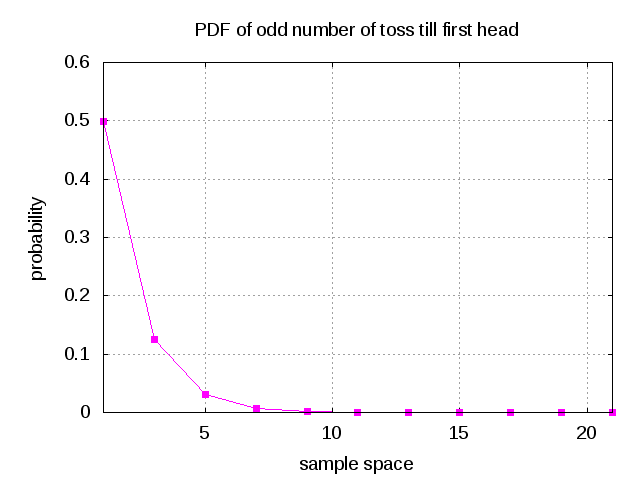# GATE-2012 ECE Q36 (math)Question 36 on math from GATE (Graduate Aptitude Test in Engineering) 2012 Electronics and Communication Engineering paper.

## Q36. A fair coin is tossed till a head appears for the first time. The probability that the number of required tosses is odd, is

(A) 1/3

(B) 1/2

(C) 2/3

(D) 3/4## Solution

Let us start by finding the sample space. The possible sample space with odd number of tosses till a head appears for the first time is,

Given that the coin is fair,

.

The total probability is,

Using Taylor series,

.

Substituting,

Matlab/Octave example

Felt it would be nice to get similar results using a simple simulation model

% Script to find the probability that odd number of tosses
% are required to get a head for the first time

clear all; close all;
N = 5*10^5;

% HEAD = 0, TAIL = 1;
% definfing the pattern corresponding to odd tosses
% till the first head - limiting to max of 21 tosses
% converted to integer (for easy comparison)
pattern_v = [ ...
sum(2.^0         .*  [0 ]);                      ... % H
sum(2.^(2:-1:0)  .*  [1 1 0 ]);                  ... % TTH
sum(2.^(4:-1:0)  .*  [1 1 1 1 0 ]);              ... % TTTTH
sum(2.^(6:-1:0)  .*  [1 1 1 1 1 1 0 ]);          ... % TTTTTTH
sum(2.^(8:-1:0)  .*  [1 1 1 1 1 1 1 1 0 ]);      ... % TTTTTTTTH ...
sum(2.^(10:-1:0) .*  [1 1 1 1 1 1 1 1 1 1 0 ]);  ...
sum(2.^(12:-1:0) .*  [1 1 1 1 1 1 1 1 1 1 1 1 0 ]); ...
sum(2.^(14:-1:0) .*  [1 1 1 1 1 1 1 1 1 1 1 1 1 1 0 ]); ...
sum(2.^(16:-1:0) .*  [1 1 1 1 1 1 1 1 1 1 1 1 1 1 1 1 0 ]); ...
sum(2.^(18:-1:0) .*  [1 1 1 1 1 1 1 1 1 1 1 1 1 1 1 1 1 1 0 ]); ...
sum(2.^(20:-1:0) .*  [1 1 1 1 1 1 1 1 1 1 1 1 1 1 1 1 1 1 1 1 0 ]); ...
];

% finding the probability for each event in the  sample space
event_v = [1:2:21];
for ii=1:length(event_v)
kk           = event_v(ii);
x            = rand(N,kk)>0.5;
xVal         = sum((ones(N,1)*[2.^([kk-1:-1:0])]).*x,2);
matchCnt(ii) = size(find(xVal==pattern_v(ii)),1);
end
totalProb = sum(matchCnt./N)Figure : Probability of odd number of tosses till first head

Based on the above, the right choice is (C) 2/3.

## References

 GATE Examination Question Papers [Previous Years] from Indian Institute of Technology, Madras http://gate.iitm.ac.in/gateqps/2012/ec.pdf

 Wiki entry on Taylor series http://en.wikipedia.org/wiki/Taylor_series